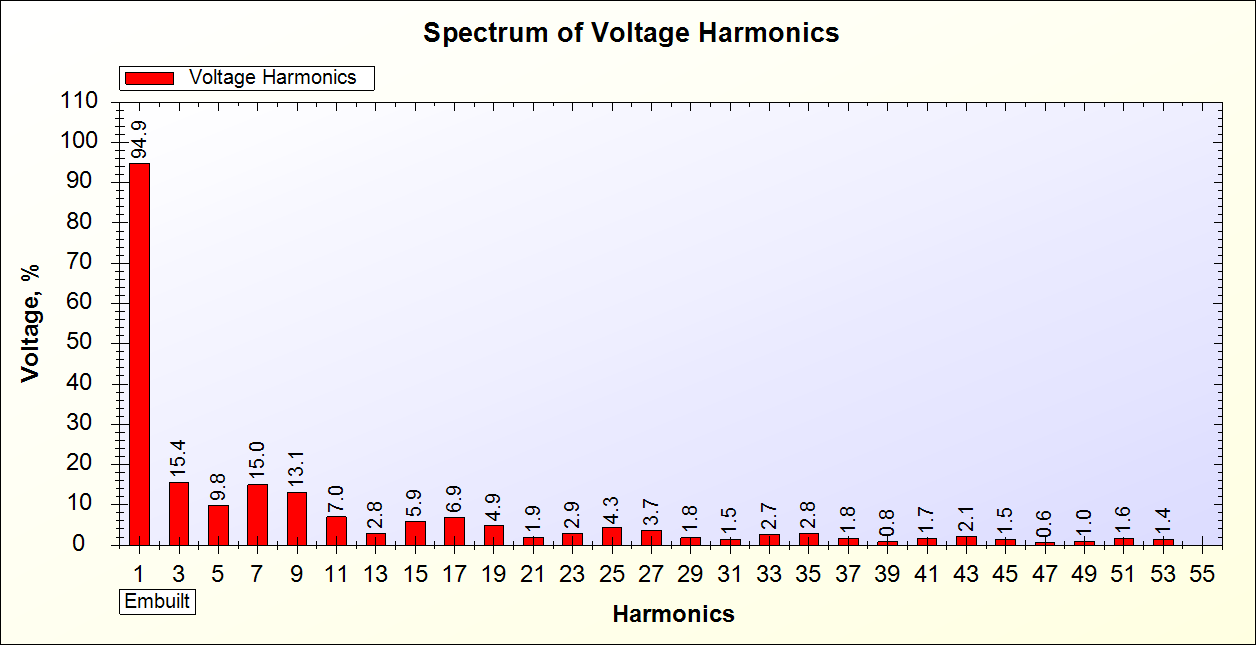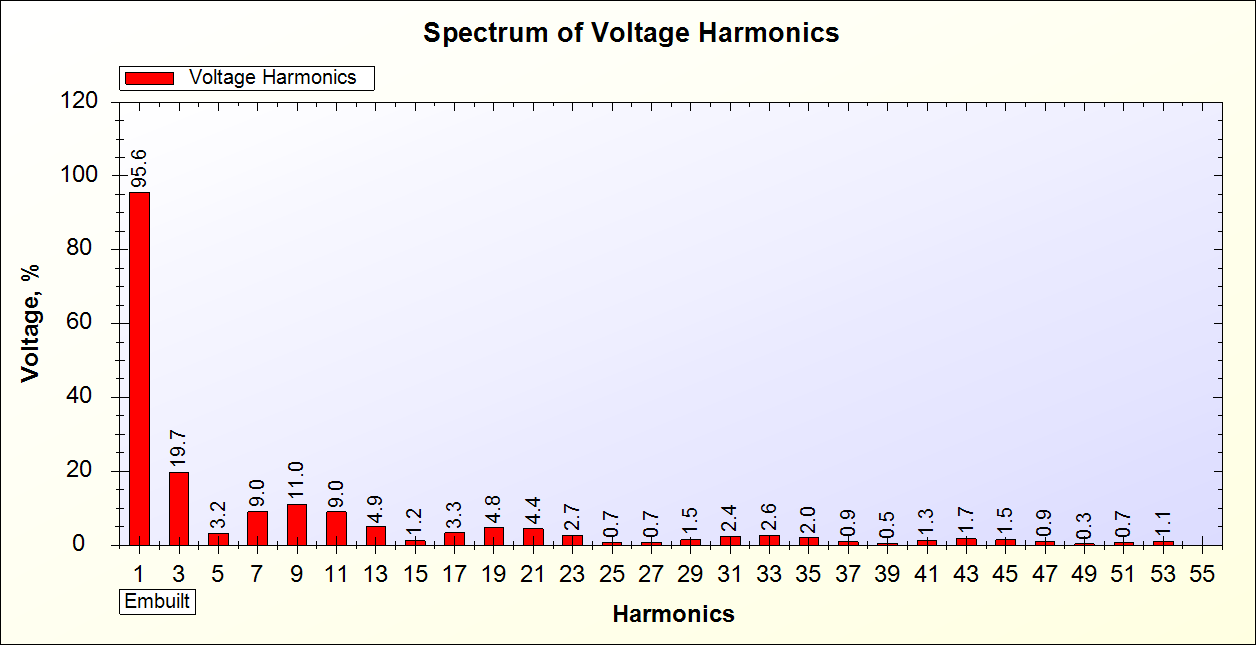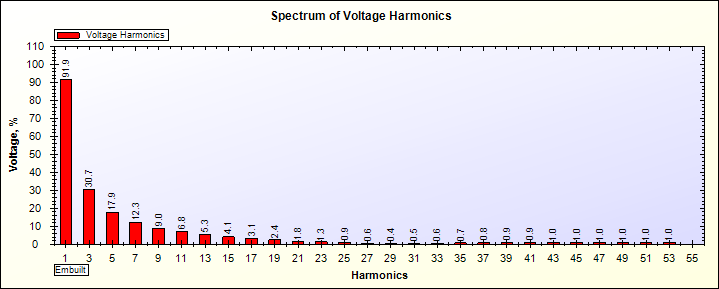# Square Wave UPS

In starting square wave UPS are made. The main problem with square wave UPS are many equipements can not run on this type of UPS. This is beacuse of equipements are made to be run on Sinewave supply.

Thus developement of Sine Wave inverter has started.

We can check the output wave form by harmonics testing. Square wave UPS produces more harmonics than sine wave.

Below is the harmonic analysis of square wave UPS.

UPS Harmonic Test Report

Date : 25-11-2014

 Standard UL1778 article 49 Harmonic Distortion Test Instrument Under Test Square Wave UPS Company Name Not Disclosed Rated Voltage and Load 12 Volt, 50A Battery Type LeadAcid Test Condition On battery source (Assuming full charged battery source)

 Load Condition Voltage Voltage Harmonics 37.685 VA 219.1 V 33.34%Notes–

1. THD is 33.4% .
2. First Harmonic is 94.9 % of Vrms.
3. Other harmonics are also high.
4. 3rd harmonic is 15.4% of Vrms.
5. 5th harmonic is lower than 3rd  .
6. 7th harmonic is also high and 15% of Vrms.
7. 9th harmonic is 13.1% of Vrms.
8. 11th ,15th and 17th harmonics are also greater than 5%.

 Load Condition Voltage Voltage Harmonics 91.206 VA 213.1 V 30.67%Notes–

1. THD is 30.67 %.
2. First Harmonic is 95.6%of Vrms.
3. Voltage is decreased by 6 Volts.
4. 3rd harmonic is increased to 19.7%
5. 5th harmonic is reduced to 3.2%.
6. 7th harmonic is also reduced to 9%.
7. 9th harmonic is reduced to 11%.
8. 11th harmonic is increased to 9.0%.
9. Other harmonics are less than 5%.

 Load Condition Voltage Voltage Harmonics 151.98 VA 190.7 V 43.08%Notes-

1. THD is 43.08%.
2. First Harmonic is 91.9 %.
3. Voltage is decreased by 23 Volts.
4. 3rd harmonic is increased to 30.7%.
5. 5th harmonic is increased to 17.9%.
6. 7th harmonic is increased to 12.3%.
7. 9th harmonic is reduced to 9%.
8. 11th harmonic is reduced to 6.8 %.
9. 13th harmonic is increased to 5.3%.
10. Other harmonics are less than 5%.
11. Harmonics from 25 to 55 are less than 1%.

Summary –

1. Expected
2. On low battery THD increases.
3. THD % of square wave inverter is very high.
4. As load increases first harmonic decreases.
5. 3rd to 15th harmonics are affected most.
6. Other harmonics are less than 2%.

Testing Instrument – SPEA-1